### Home > A2C > Chapter 5 > Lesson 5.2.1 > Problem5-64

5-64.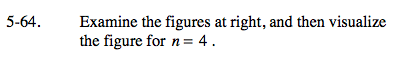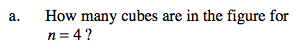Assume that cubes which are blocked from view but would complete the figure are present.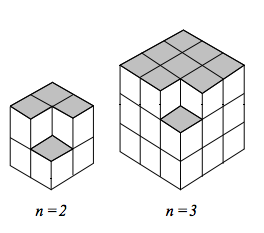The n = 2 figure is a 2 × 2 × 2 cube with one corner cube missing. What would the n = 4 figure look like?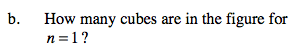Pay close attention to the position of the missing cube. Where would the missing cube be in the n = 1 figure?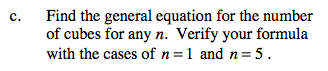Imagine that the smaller cubes have side length 1. What is the volume of each large cube?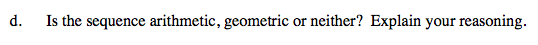Do you need to add or multiply to determine the number of cubes in the 'next' figure?